geom_path() connects the observations in the order in which they appear in the data. geom_line() connects them in order of the variable on the x axis. geom_step() creates a stairstep plot, highlighting exactly when changes occur. The group aesthetic determines which cases are connected together.

geom_path(
mapping = NULL,
data = NULL,
stat = "identity",
position = "identity",
...,
lineend = "butt",
linejoin = "round",
linemitre = 10,
arrow = NULL,
na.rm = FALSE,
show.legend = NA,
inherit.aes = TRUE
)

geom_line(
mapping = NULL,
data = NULL,
stat = "identity",
position = "identity",
na.rm = FALSE,
orientation = NA,
show.legend = NA,
inherit.aes = TRUE,
...
)

geom_step(
mapping = NULL,
data = NULL,
stat = "identity",
position = "identity",
direction = "hv",
na.rm = FALSE,
show.legend = NA,
inherit.aes = TRUE,
...
)

## Arguments

mapping Set of aesthetic mappings created by aes() or aes_(). If specified and inherit.aes = TRUE (the default), it is combined with the default mapping at the top level of the plot. You must supply mapping if there is no plot mapping. The data to be displayed in this layer. There are three options: If NULL, the default, the data is inherited from the plot data as specified in the call to ggplot(). A data.frame, or other object, will override the plot data. All objects will be fortified to produce a data frame. See fortify() for which variables will be created. A function will be called with a single argument, the plot data. The return value must be a data.frame, and will be used as the layer data. A function can be created from a formula (e.g. ~ head(.x, 10)). The statistical transformation to use on the data for this layer, as a string. Position adjustment, either as a string, or the result of a call to a position adjustment function. Other arguments passed on to layer(). These are often aesthetics, used to set an aesthetic to a fixed value, like colour = "red" or size = 3. They may also be parameters to the paired geom/stat. Line end style (round, butt, square). Line join style (round, mitre, bevel). Line mitre limit (number greater than 1). Arrow specification, as created by grid::arrow(). If FALSE, the default, missing values are removed with a warning. If TRUE, missing values are silently removed. logical. Should this layer be included in the legends? NA, the default, includes if any aesthetics are mapped. FALSE never includes, and TRUE always includes. It can also be a named logical vector to finely select the aesthetics to display. If FALSE, overrides the default aesthetics, rather than combining with them. This is most useful for helper functions that define both data and aesthetics and shouldn't inherit behaviour from the default plot specification, e.g. borders(). The orientation of the layer. The default (NA) automatically determines the orientation from the aesthetic mapping. In the rare event that this fails it can be given explicitly by setting orientation to either "x" or "y". See the Orientation section for more detail. direction of stairs: 'vh' for vertical then horizontal, 'hv' for horizontal then vertical, or 'mid' for step half-way between adjacent x-values.

## Details

An alternative parameterisation is geom_segment(), where each line corresponds to a single case which provides the start and end coordinates.

## Orientation

This geom treats each axis differently and, thus, can thus have two orientations. Often the orientation is easy to deduce from a combination of the given mappings and the types of positional scales in use. Thus, ggplot2 will by default try to guess which orientation the layer should have. Under rare circumstances, the orientation is ambiguous and guessing may fail. In that case the orientation can be specified directly using the orientation parameter, which can be either "x" or "y". The value gives the axis that the geom should run along, "x" being the default orientation you would expect for the geom.

## Aesthetics

geom_path() understands the following aesthetics (required aesthetics are in bold):

• x

• y

• alpha

• colour

• group

• linetype

• size

Learn more about setting these aesthetics in vignette("ggplot2-specs").

## Missing value handling

geom_path(), geom_line(), and geom_step() handle NA as follows:

• If an NA occurs in the middle of a line, it breaks the line. No warning is shown, regardless of whether na.rm is TRUE or FALSE.

• If an NA occurs at the start or the end of the line and na.rm is FALSE (default), the NA is removed with a warning.

• If an NA occurs at the start or the end of the line and na.rm is TRUE, the NA is removed silently, without warning.

geom_polygon(): Filled paths (polygons); geom_segment(): Line segments

## Examples

# geom_line() is suitable for time series
ggplot(economics, aes(date, unemploy)) + geom_line()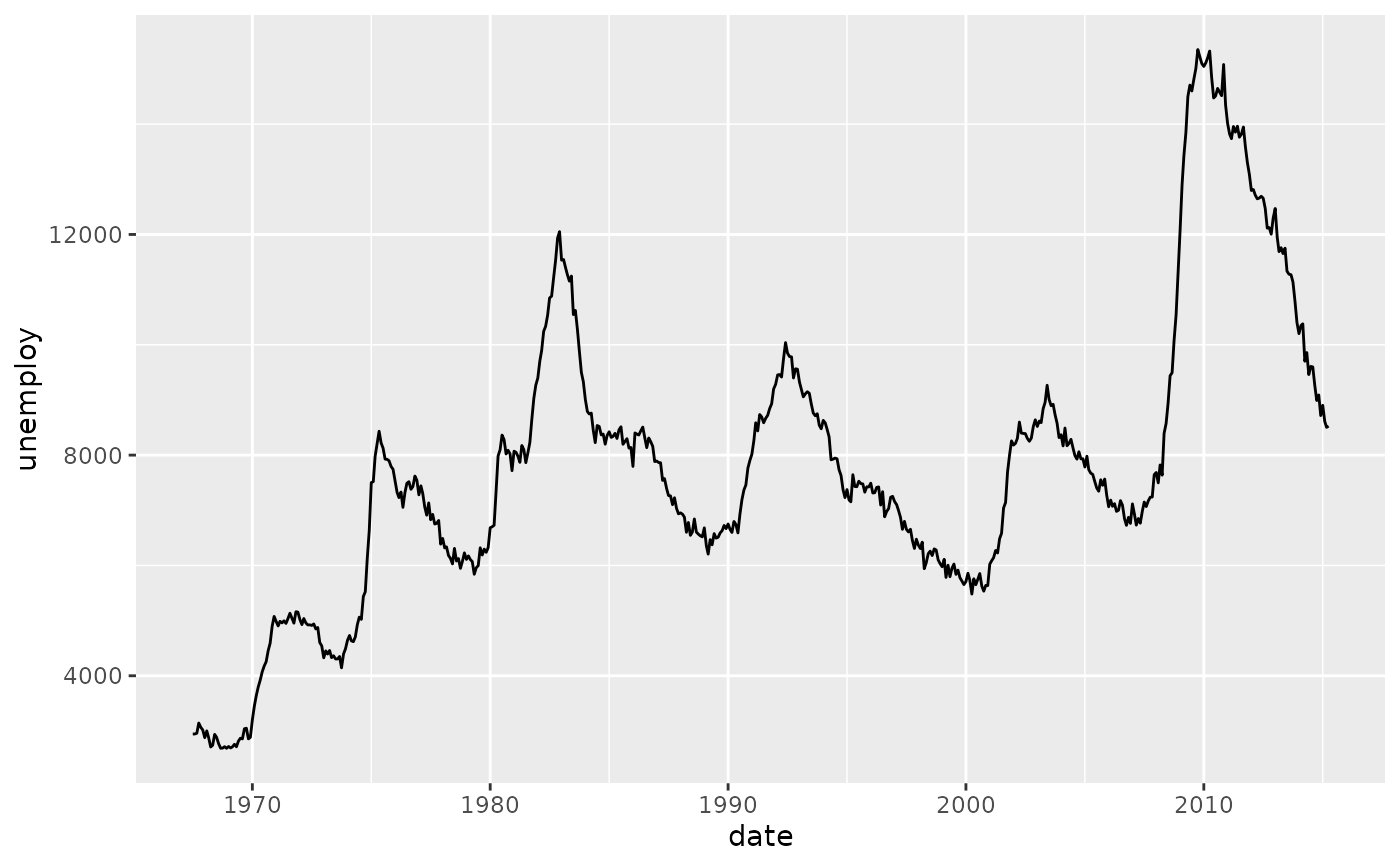ggplot(economics_long, aes(date, value01, colour = variable)) +
geom_line()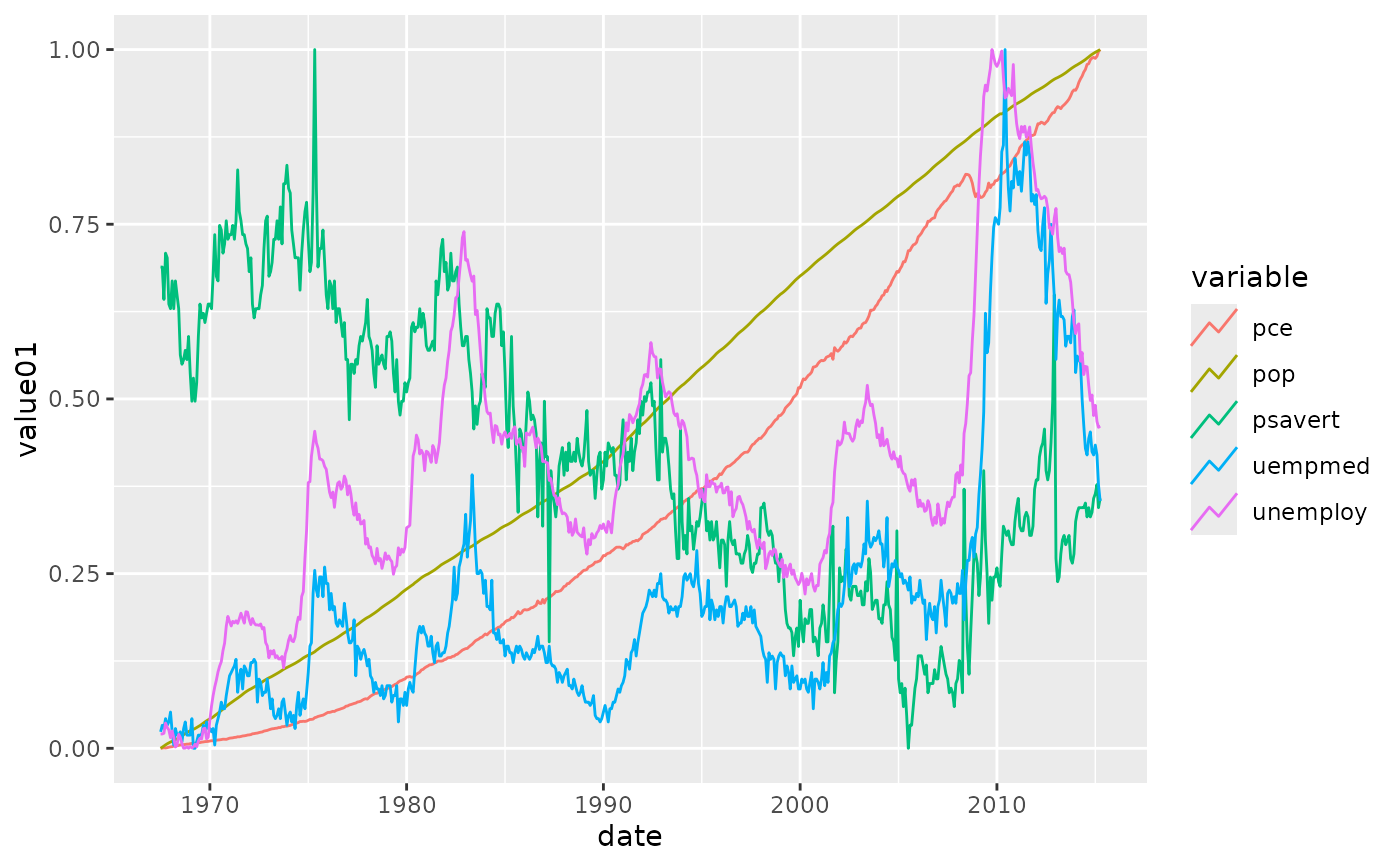# You can get a timeseries that run vertically by setting the orientation
ggplot(economics, aes(unemploy, date)) + geom_line(orientation = "y")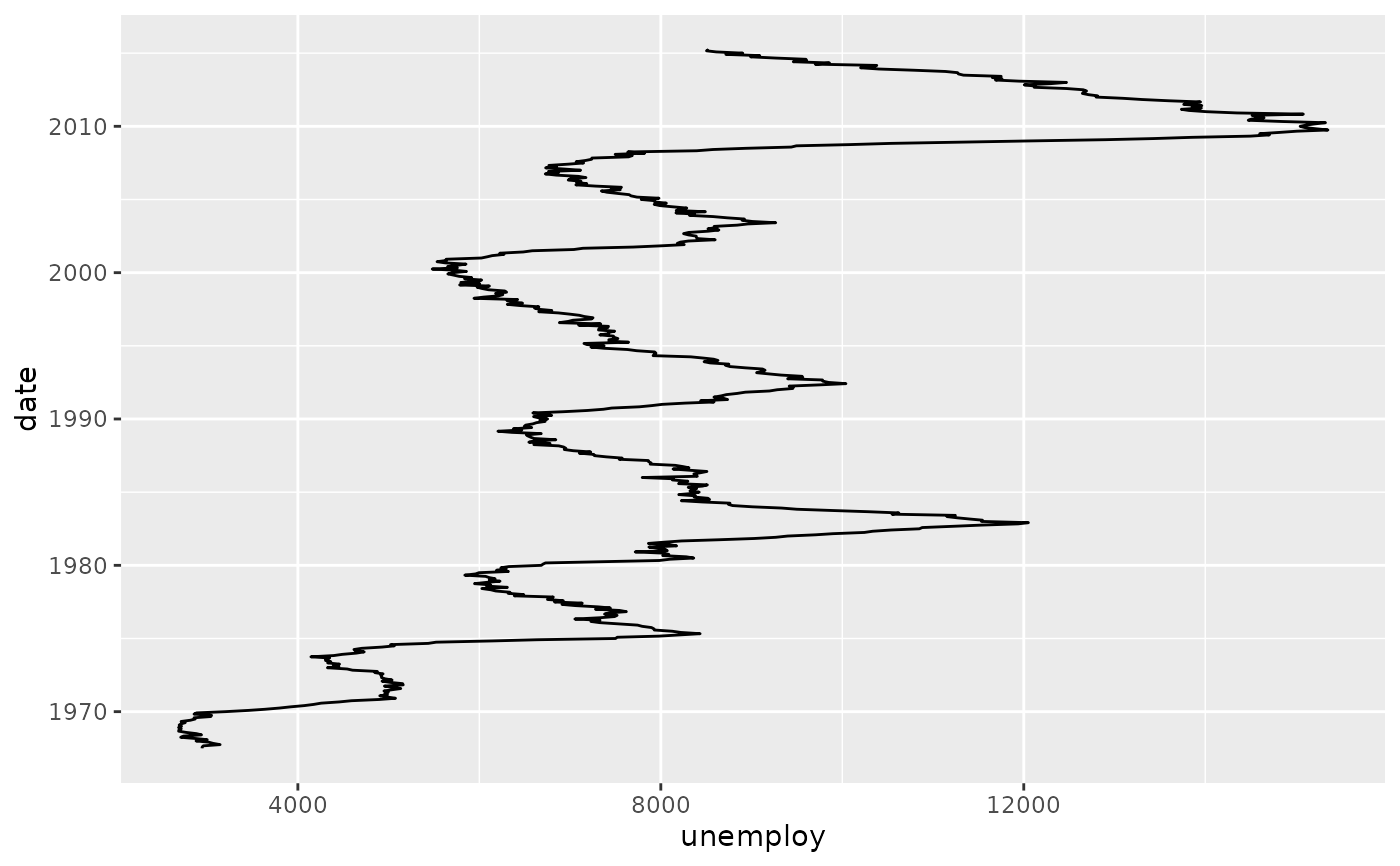# geom_step() is useful when you want to highlight exactly when
# the y value changes
recent <- economics[economics\$date > as.Date("2013-01-01"), ]
ggplot(recent, aes(date, unemploy)) + geom_line()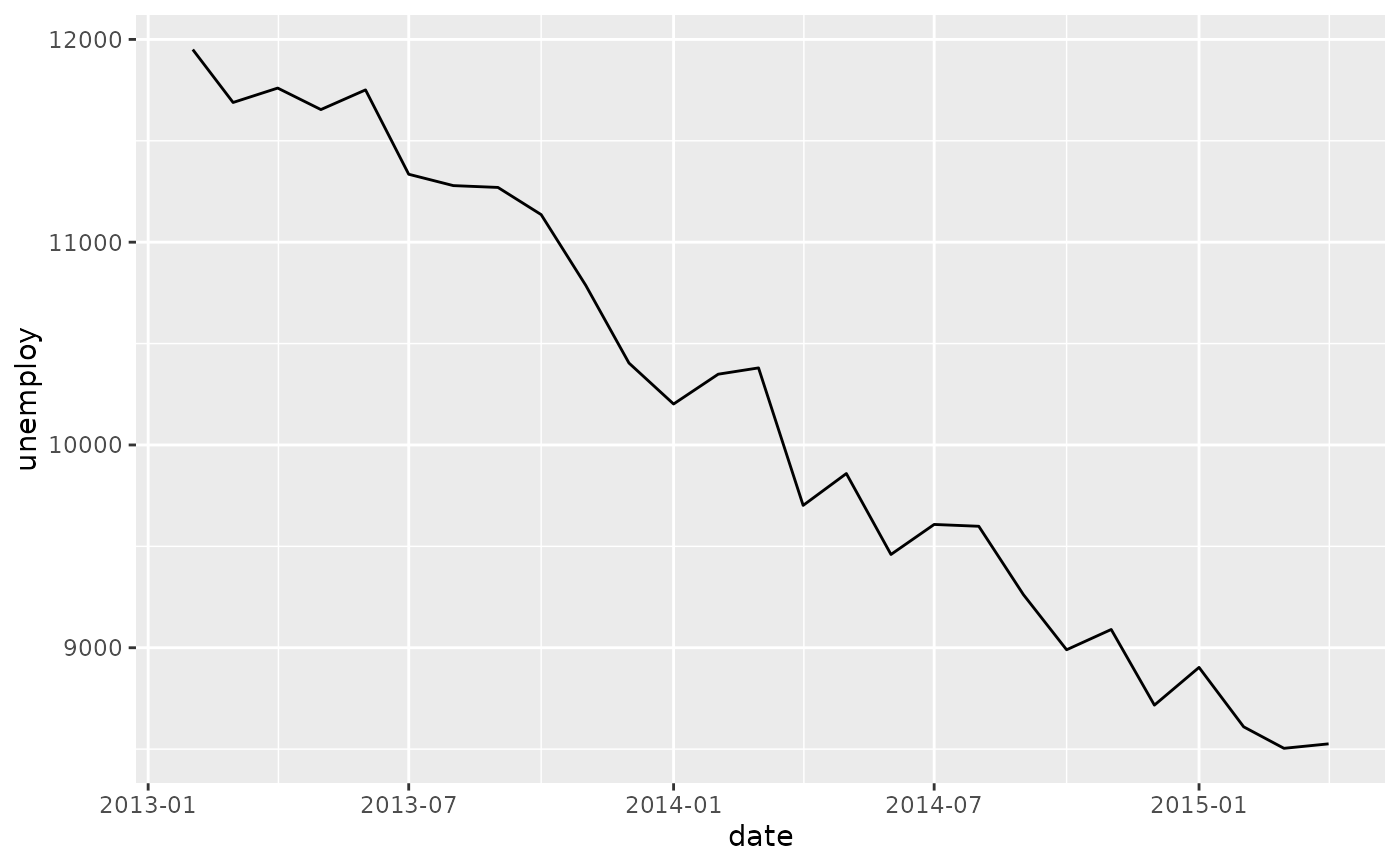ggplot(recent, aes(date, unemploy)) + geom_step()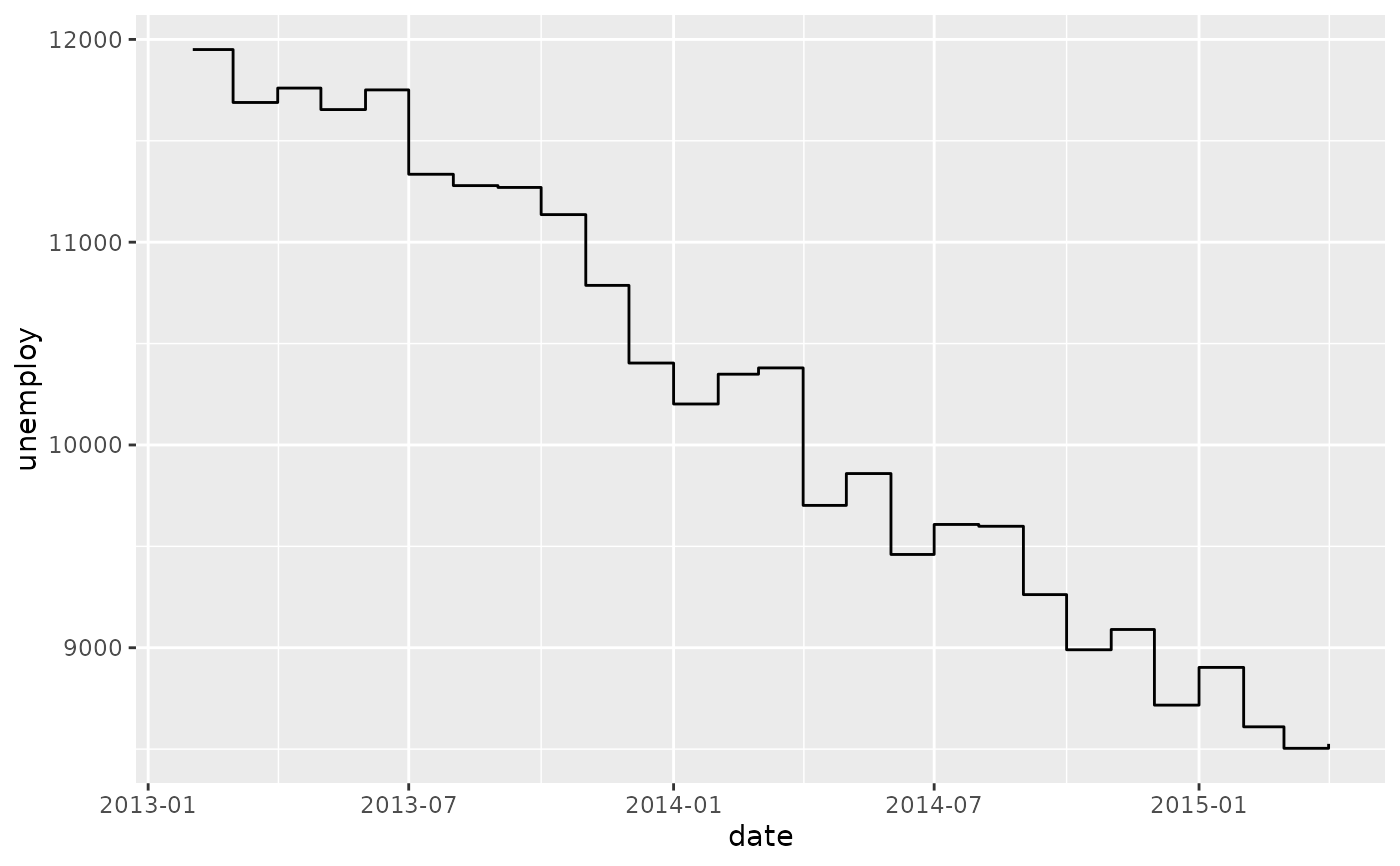# geom_path lets you explore how two variables are related over time,
# e.g. unemployment and personal savings rate
m <- ggplot(economics, aes(unemploy/pop, psavert))
m + geom_path()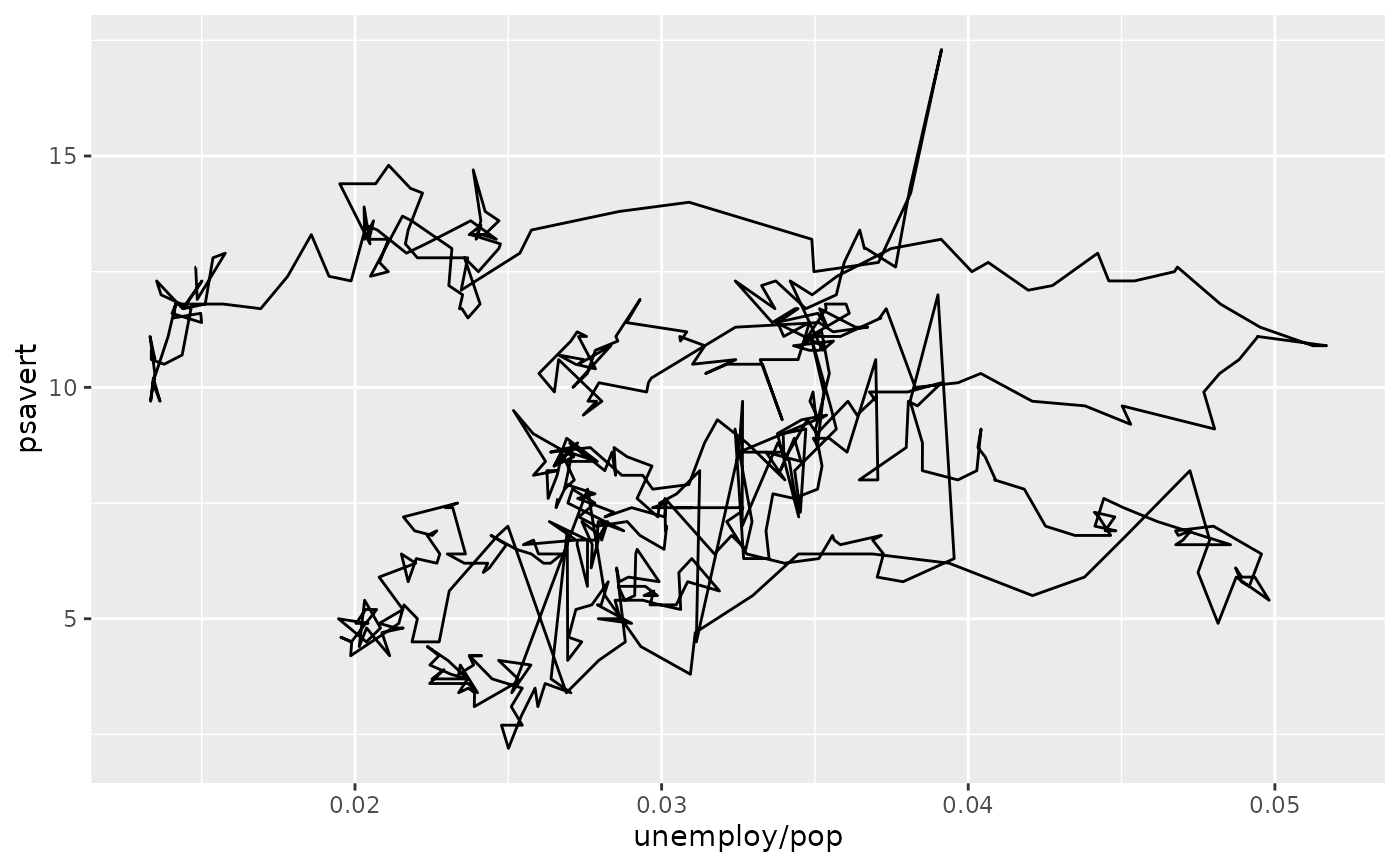m + geom_path(aes(colour = as.numeric(date)))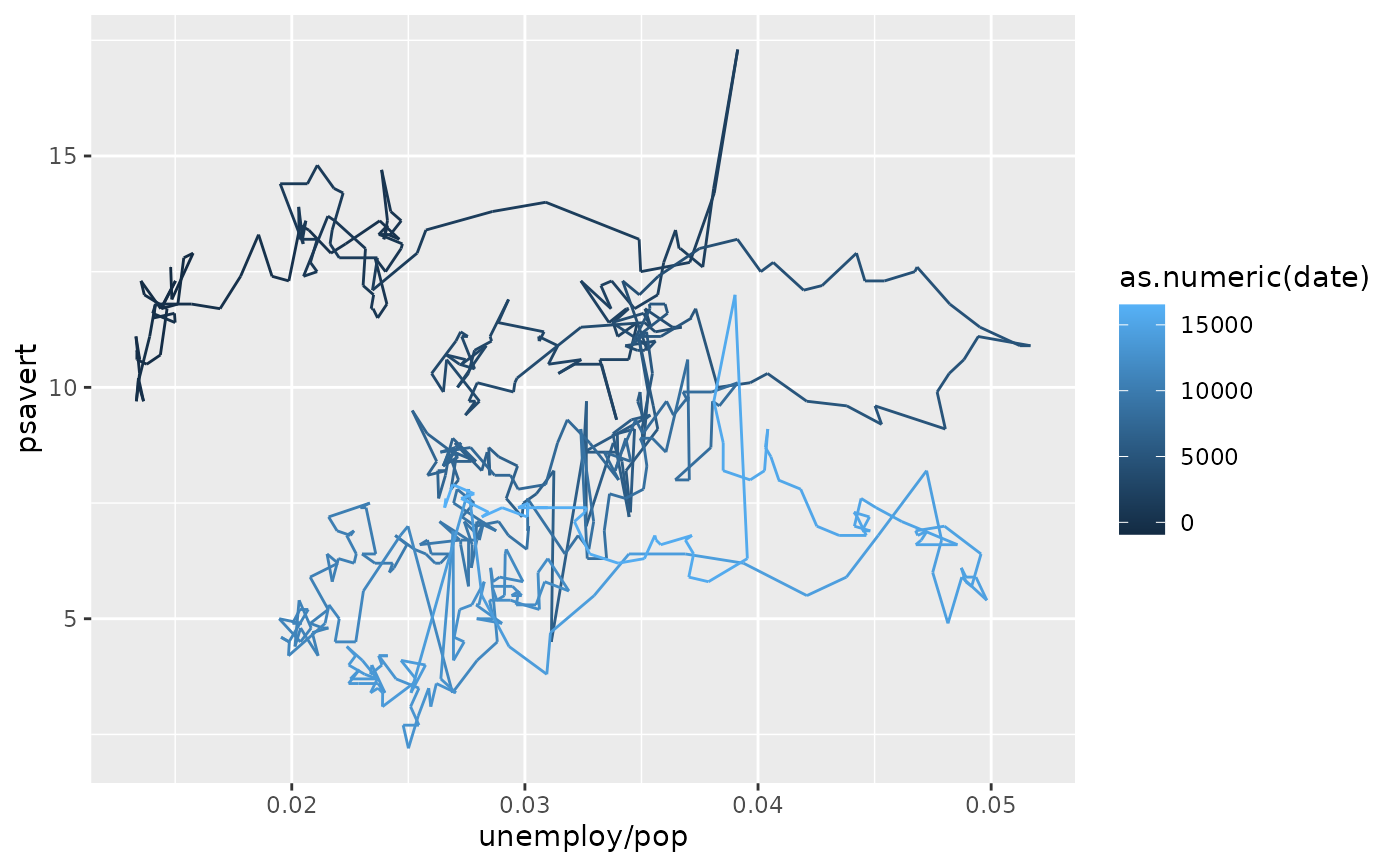# Changing parameters ----------------------------------------------
ggplot(economics, aes(date, unemploy)) +
geom_line(colour = "red")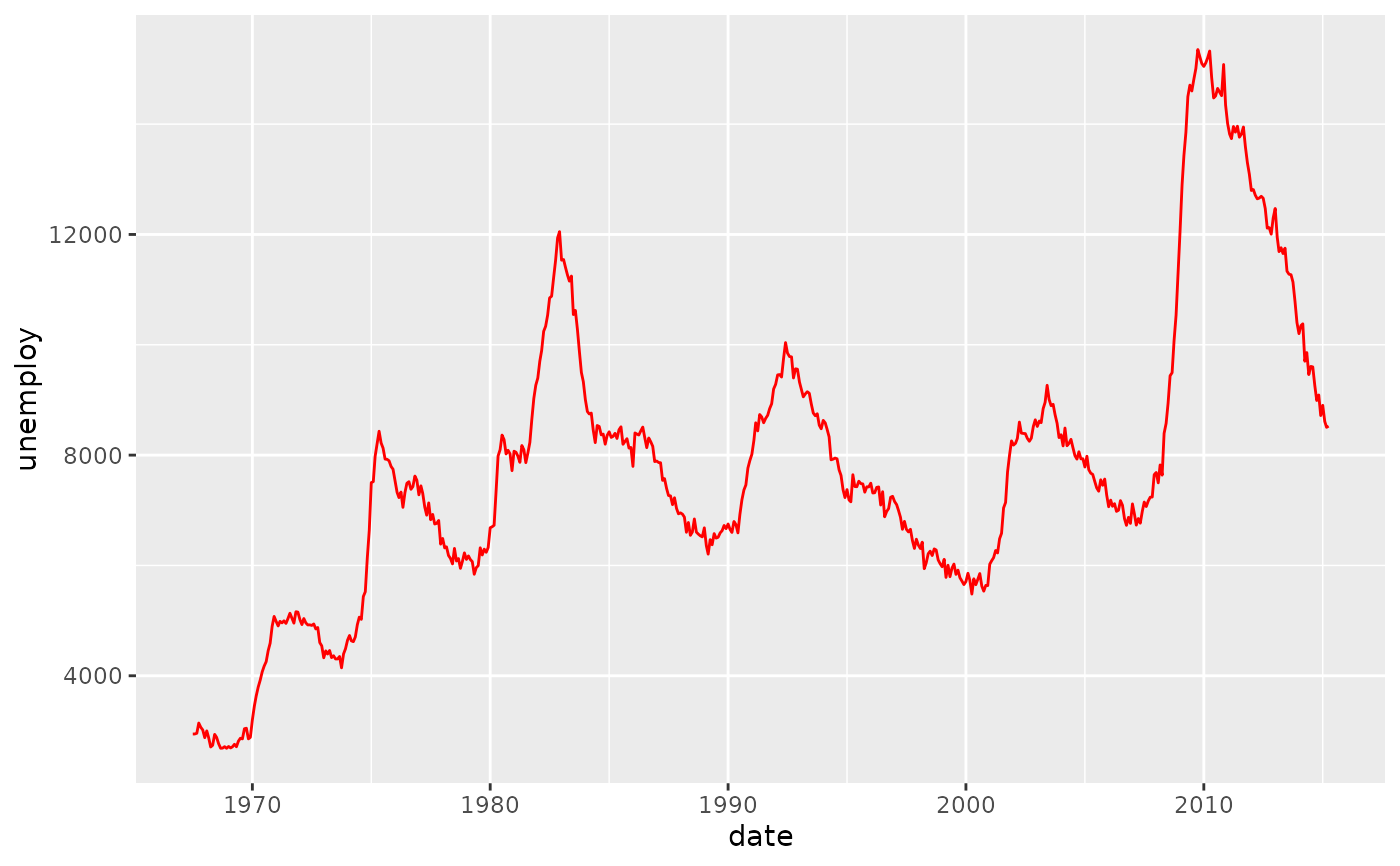# Use the arrow parameter to add an arrow to the line
# See ?arrow for more details
c <- ggplot(economics, aes(x = date, y = pop))
c + geom_line(arrow = arrow())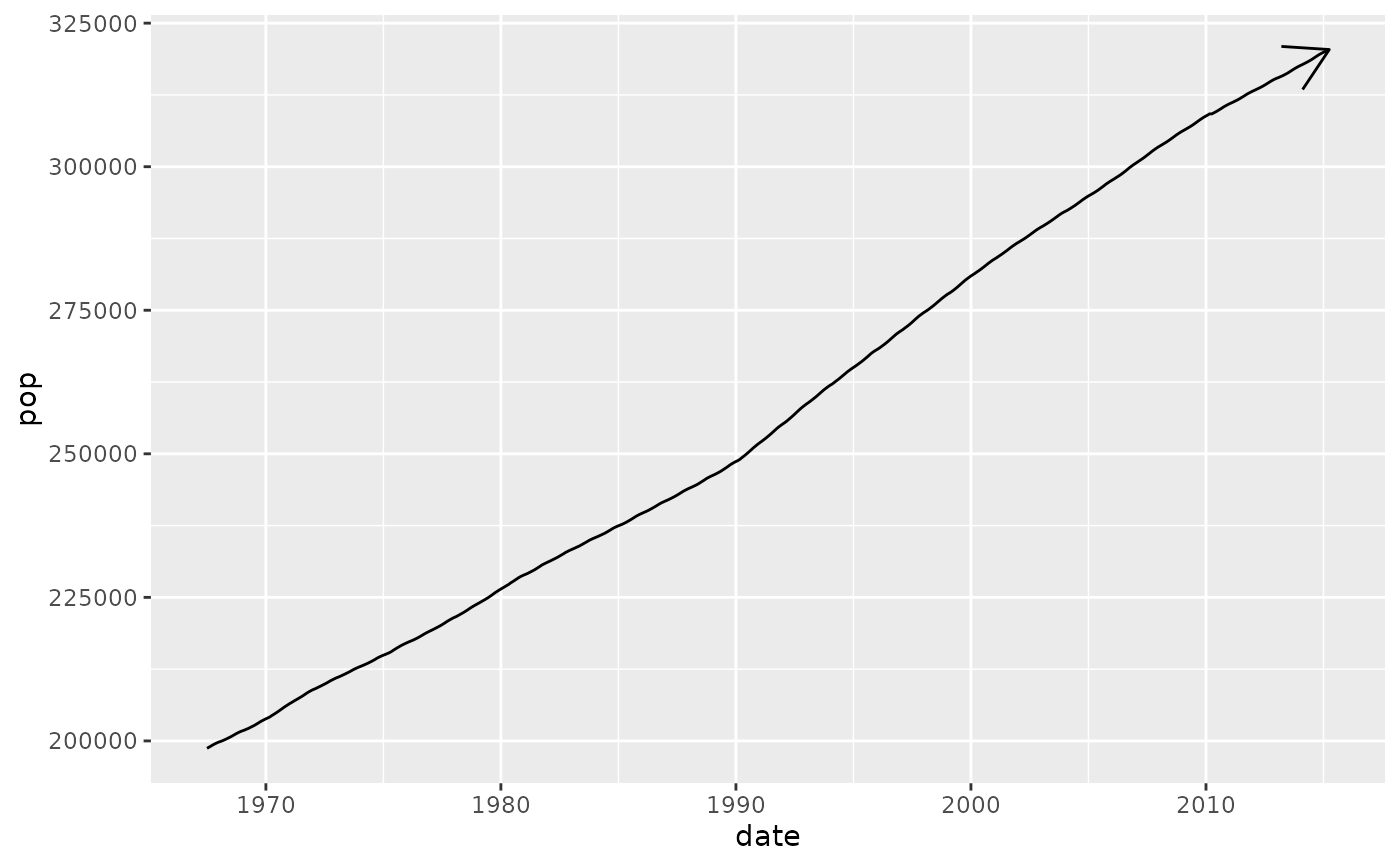c + geom_line(
arrow = arrow(angle = 15, ends = "both", type = "closed")
)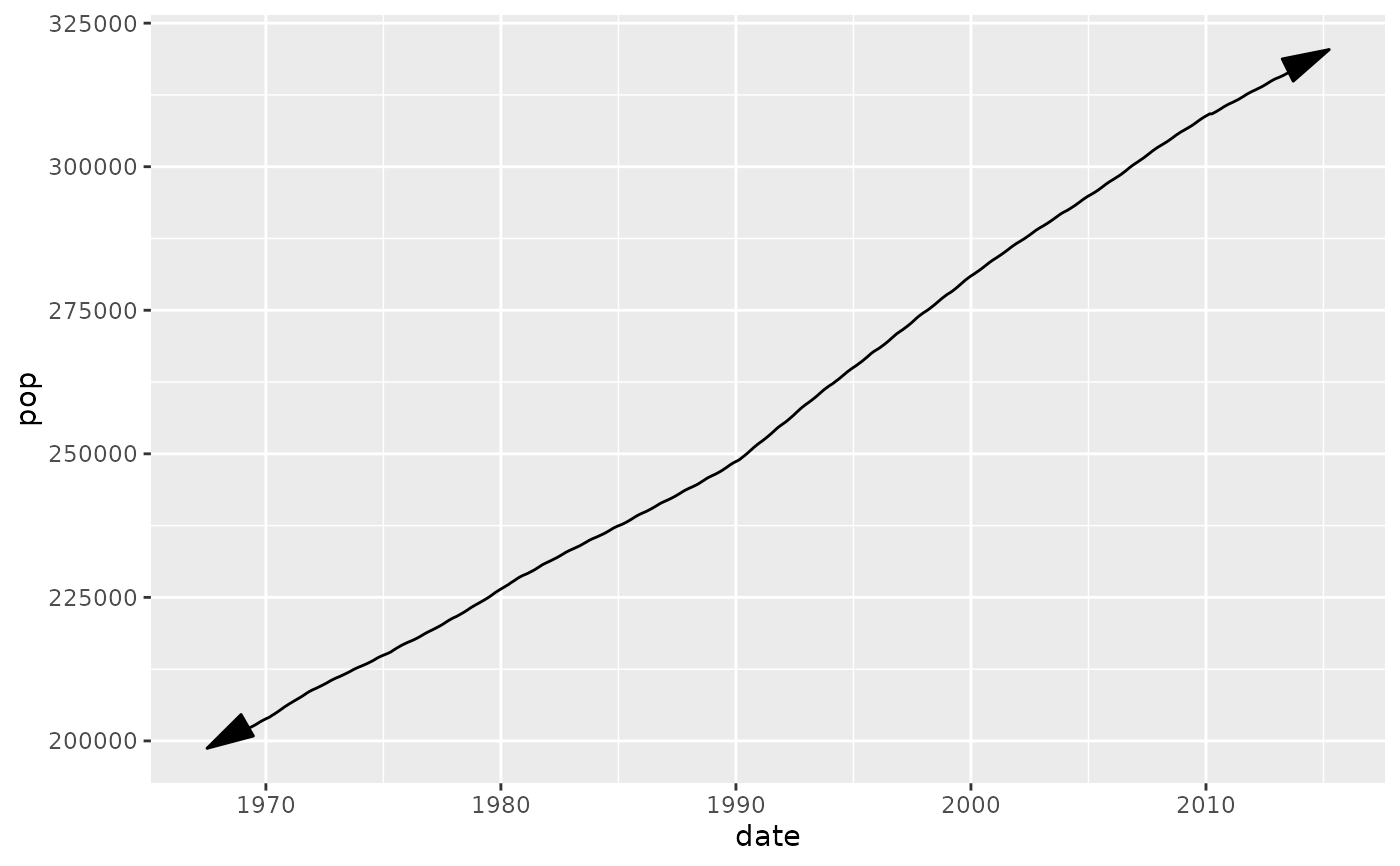# Control line join parameters
df <- data.frame(x = 1:3, y = c(4, 1, 9))
base <- ggplot(df, aes(x, y))
base + geom_path(size = 10)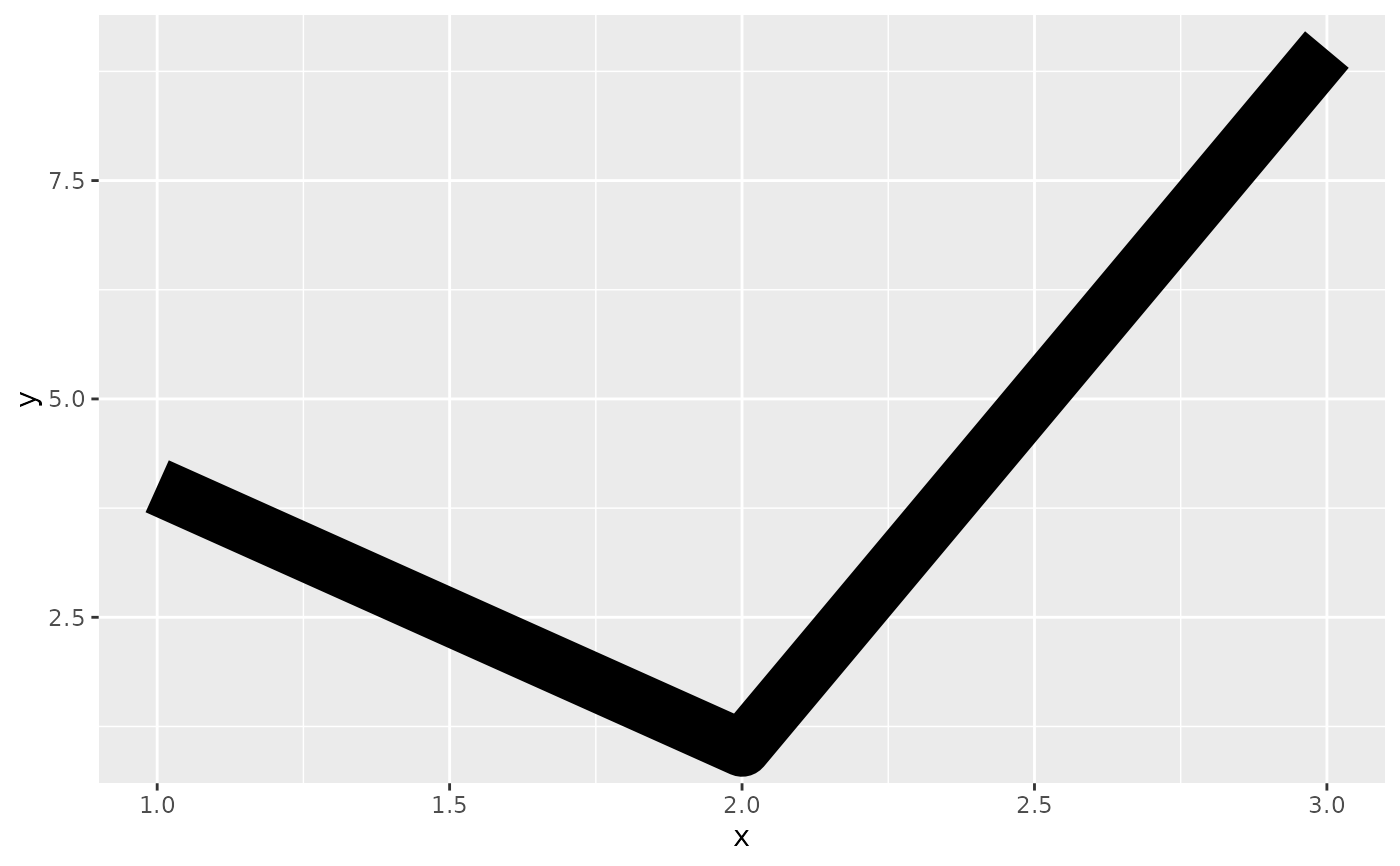base + geom_path(size = 10, lineend = "round")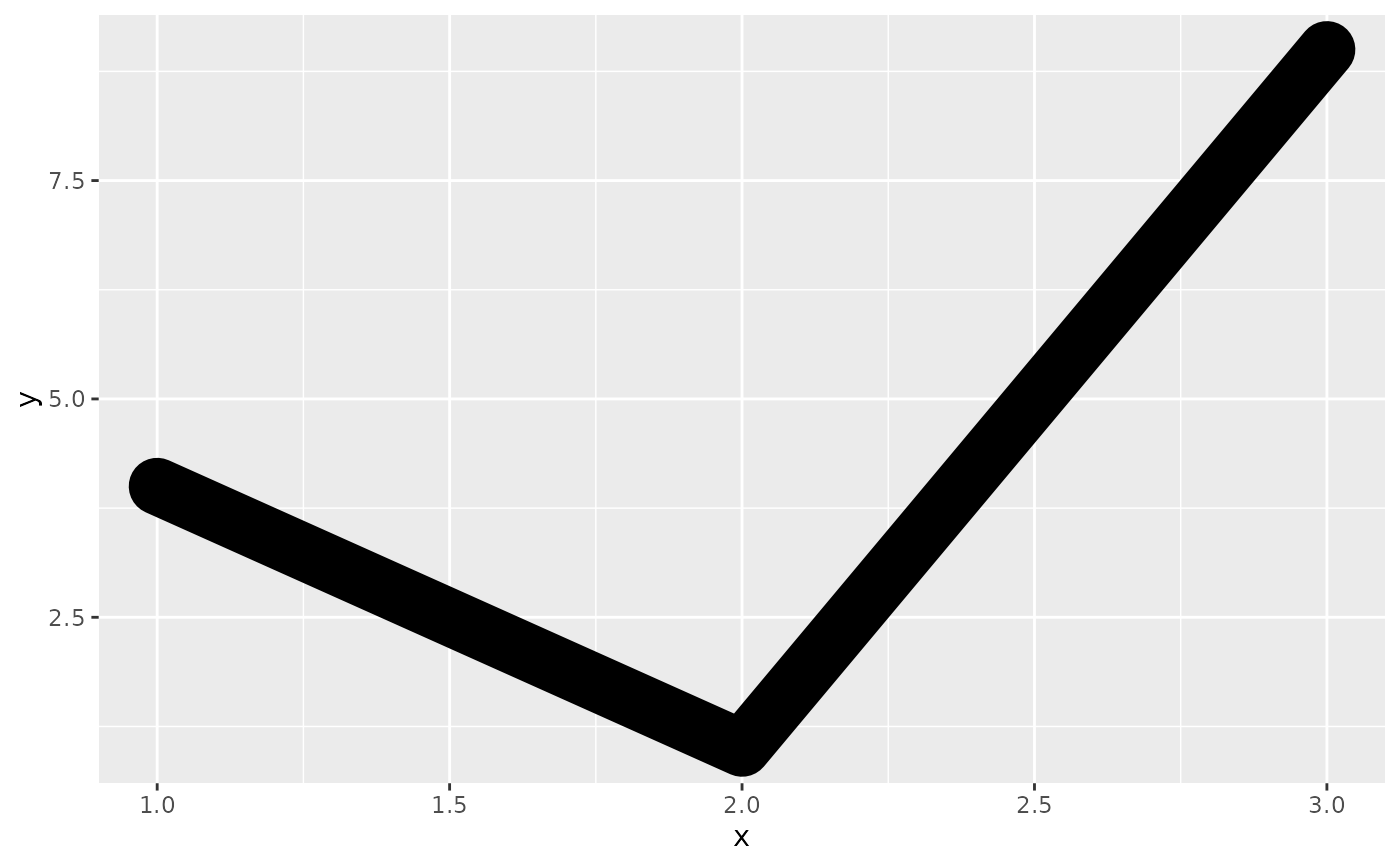base + geom_path(size = 10, linejoin = "mitre", lineend = "butt")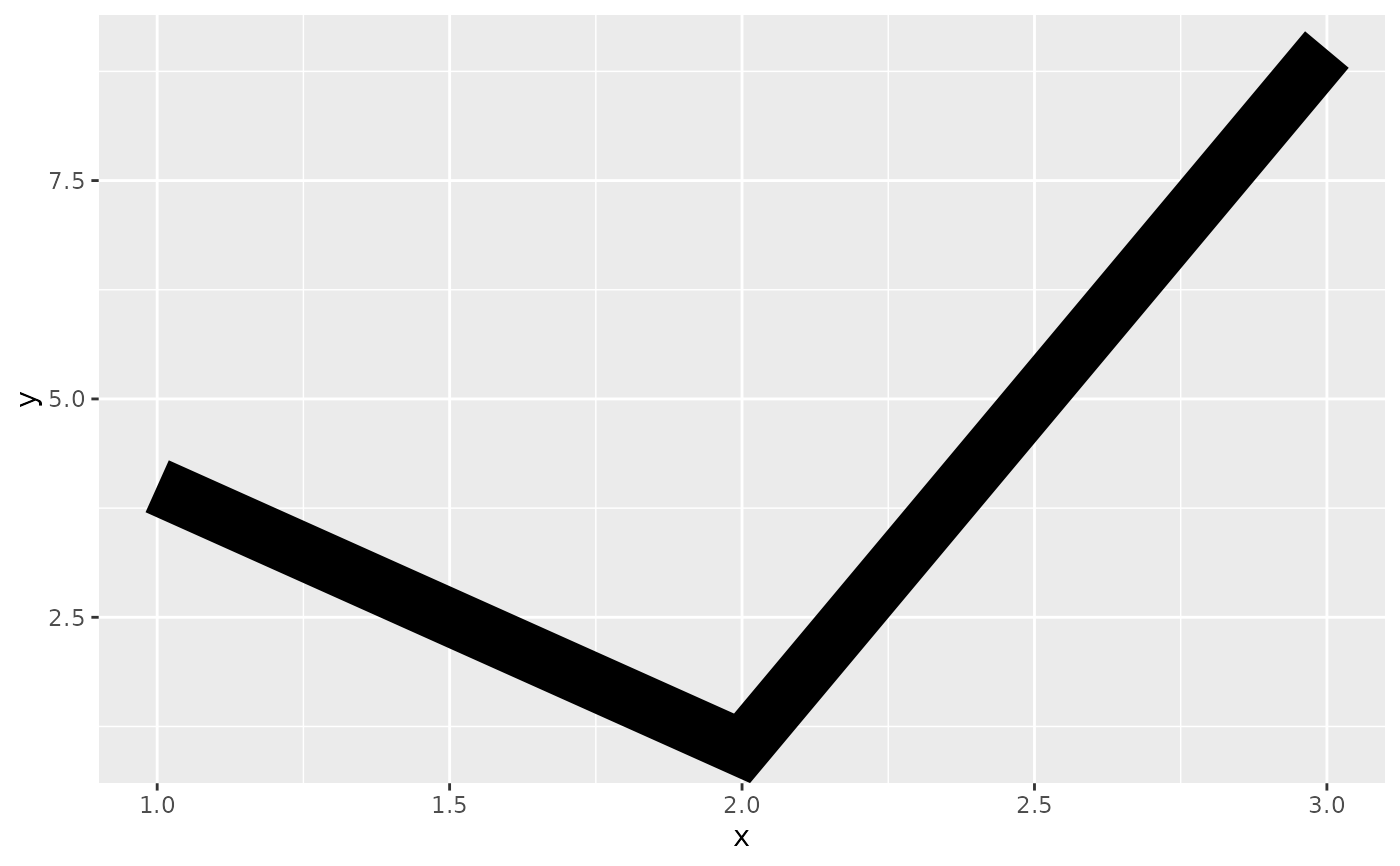# You can use NAs to break the line.
df <- data.frame(x = 1:5, y = c(1, 2, NA, 4, 5))
ggplot(df, aes(x, y)) + geom_point() + geom_line()
#> Warning: Removed 1 rows containing missing values (geom_point).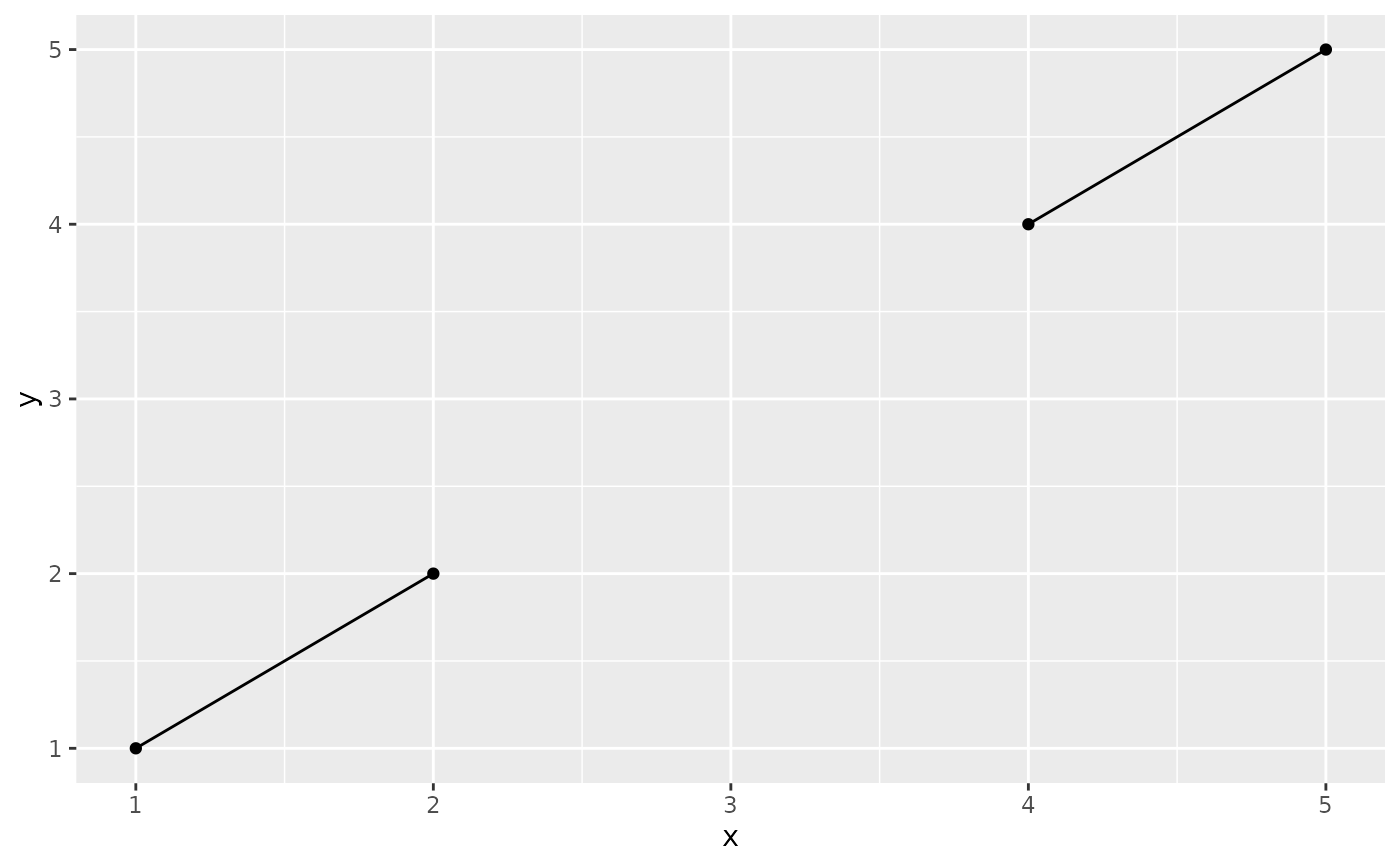# \donttest{
# Setting line type vs colour/size
# Line type needs to be applied to a line as a whole, so it can
# not be used with colour or size that vary across a line
x <- seq(0.01, .99, length.out = 100)
df <- data.frame(
x = rep(x, 2),
y = c(qlogis(x), 2 * qlogis(x)),
group = rep(c("a","b"),
each = 100)
)
p <- ggplot(df, aes(x=x, y=y, group=group))
# These work
p + geom_line(linetype = 2)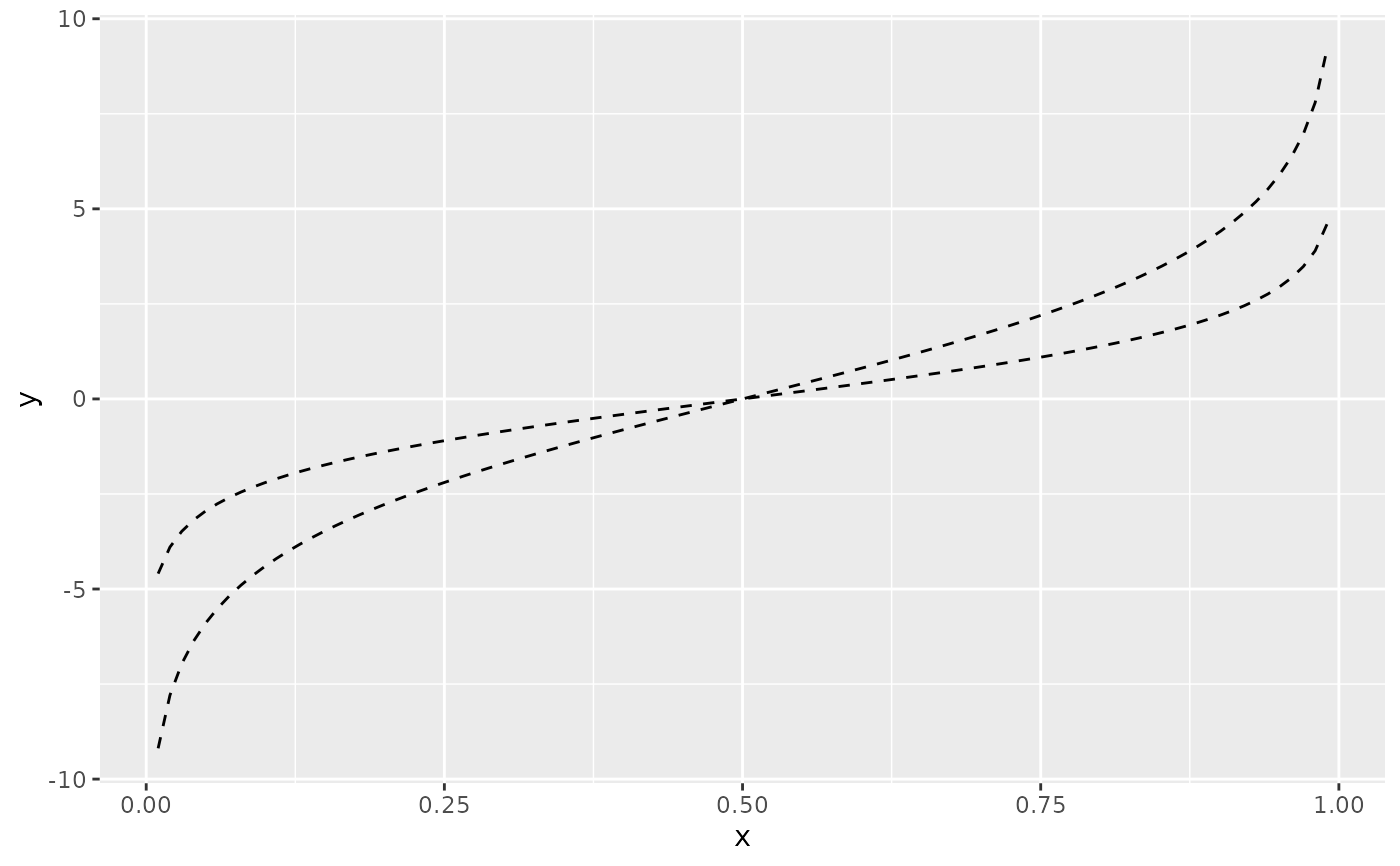p + geom_line(aes(colour = group), linetype = 2)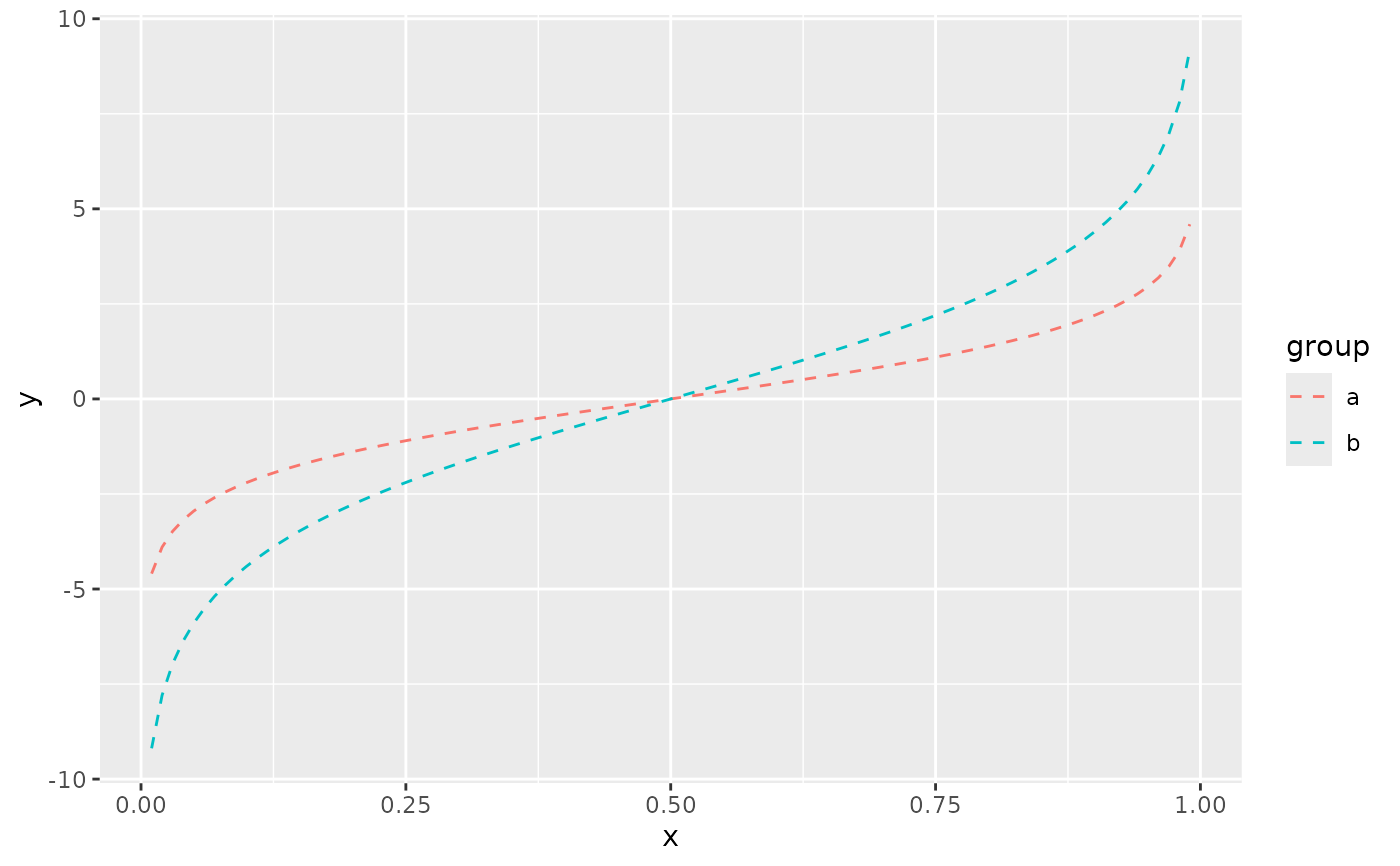p + geom_line(aes(colour = x))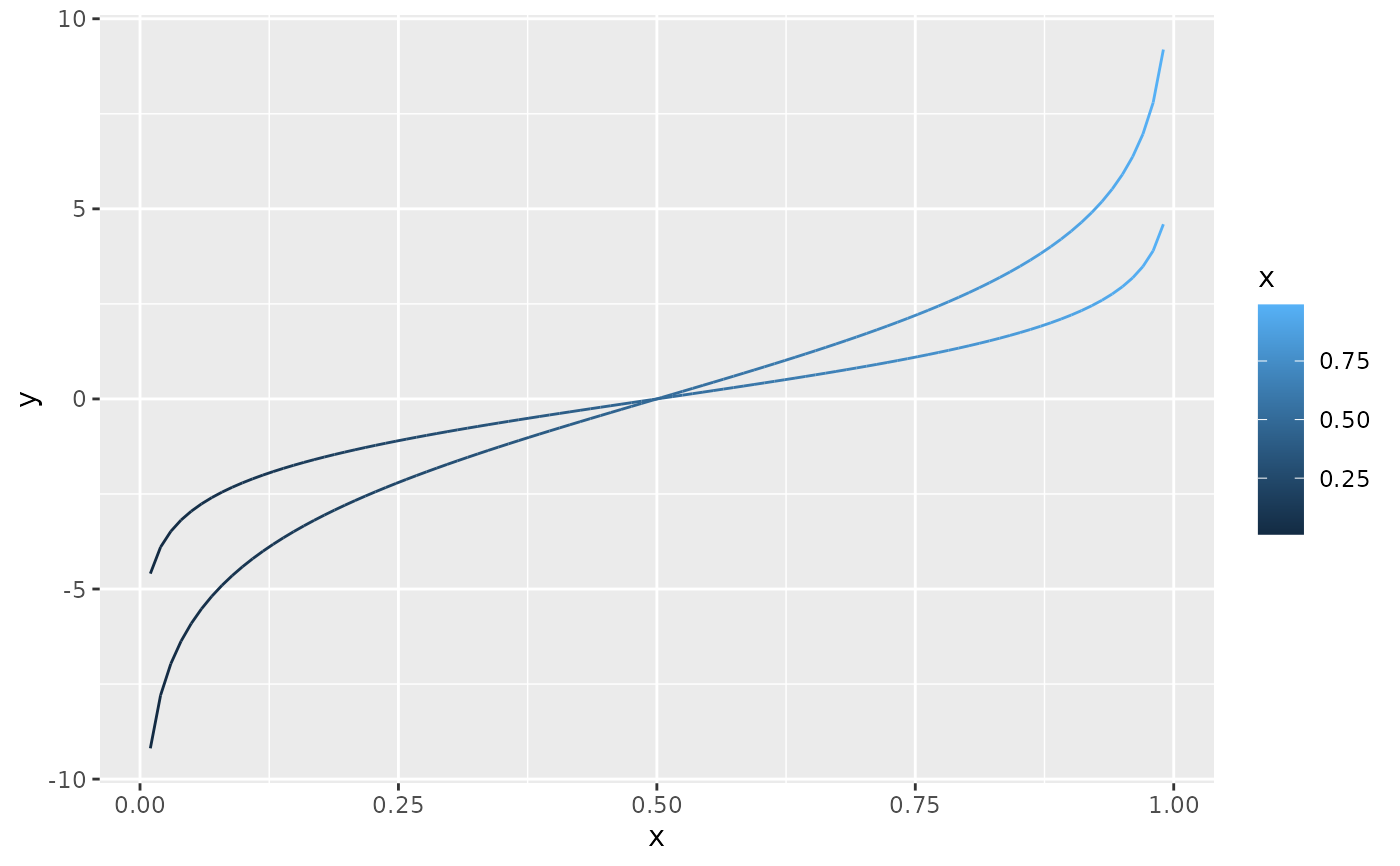# But this doesn't
should_stop(p + geom_line(aes(colour = x), linetype=2))# }